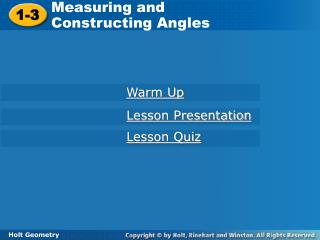DownloadDownload Presentation1-3

# 1-3

Download Presentation## 1-3

- - - - - - - - - - - - - - - - - - - - - - - - - - - E N D - - - - - - - - - - - - - - - - - - - - - - - - - - -
##### Presentation Transcript

1. Measuring and Constructing Angles 1-3 Warm Up Lesson Presentation Lesson Quiz Holt Geometry

2. Are you ready? • 1) Draw AB and AC, where A, B, and C are noncollinear. • 2) Draw opposite rays DE and DF. • Solve each equation. • 2x + 3 + x – 4 + 3x – 5 = 180 • 4)5x + 2 = 8x – 10 31

3. Objectives TSW name and classify angles. TSW measure and construct angles and angle bisectors.

4. Surveyors use angles to help them measure and map the earth’s surface.

5. Vocabulary angle right angle vertex obtuse angle interior of an angle straight angle exterior of an angle congruent angles measure angle bisector degree acute angle

6. A transit is a tool for measuring angles. It consists of a telescope that swivels horizontally and vertically. Using a transit, a survey or can measure the angle formed by his or her location and two distant points. An angleis a figure formed by two rays, or sides, with a common endpoint called the vertex(plural: vertices). You can name an angle several ways: by its vertex, by a point on each ray and the vertex, or by a number.

7. The set of all points between the sides of the angle is the interior of an angle. The exterior of an angleis the set of all points outside the angle. Angle Name R, SRT, TRS, or 1 You cannot name an angle just by its vertex if the point is the vertex of more than one angle. In this case, you must use all three points to name the angle, and the middle point is always the vertex.

8. Example 1: Naming Angles A surveyor recorded the angles formed by a transit (point A) and three distant points, B, C, and D. Name three of the angles. Possible answer:

9. Example 2 Write the different ways you can name the angles in the diagram.

10. The measureof an angle is usually given in degrees. Since there are 360° in a circle, one degreeis of a circle. When you use a protractor to measure angles, you are applying the following postulate.

11. If OC corresponds with c and OD corresponds with d, mDOC = |d– c| or |c– d|. You can use the Protractor Postulate to help you classify angles by their measure. The measure of an angle is the absolute value of the difference of the real numbers that the rays correspond with on a protractor.

12. Example 3: Measuring and Classifying Angles Find the measure of each angle. Then classify each as acute, right, or obtuse. YXZ: ZXV: YXW: ZXW: WXV:

13. Example 4 Use the diagram to find the measure of each angle. Then classify each as acute, right, or obtuse. AOD EOC COD BOA DOB

14. Congruent angles are angles that have the same measure. In the diagram, mABC = mDEF, so you can write ABC  DEF. This is read as “angle ABC is congruent to angle DEF.”Arc marks are used to show that the two angles are congruent. The Angle Addition Postulate is very similar to the Segment Addition Postulate that you learned in the previous lesson.

15. Example 5: Using the Angle Addition Postulate mDEG = 115°, and mDEF = 48°. Find mFEG

16. Example 6 mXWZ = 121° and mXWY = 59°. Find mYWZ.

17. Example 6a mABD = 37° and m<BC = 84°. Find mDBC. Example 6b mXWZ = 121° and mXWY = 59°. Find mYWZ.

18. An angle bisector is a ray that divides an angle into two congruent angles. JK bisects LJM; thus LJKKJM.

19. KM bisects JKL, mJKM = (4x + 6)°, and mMKL = (7x – 12)°. Find mJKM. Example 7: Finding the Measure of an Angle

20. QS bisects PQR, mPQS = (5y – 1)°, and mPQR = (8y + 12)°. Find mPQS. Example 8 Find the measure of each angle.

21. JK bisects LJM, mLJK = (-10x + 3)°, and mKJM = (–x + 21)°. Find mLJM. Example 9 Find the measure of each angle.

22. Example 10 A surveyor at point S discovers that the angle between peaks A and B is 3 times as large as the angle between peaks B and C. The surveyor knows that ∠ASC is a right angle. Find m∠ASB and m∠BSC.

23. Lesson Quiz: Part I Classify each angle as acute, right, or obtuse. 1. XTS 2. WTU 3. K is in the interior of LMN, mLMK =52°, and mKMN = 12°. Find mLMN.

24. 4. BD bisects ABC, mABD = , and mDBC = (y + 4)°. Find mABC. Lesson Quiz: Part II 5. Use a protractor to draw an angle with a measure of 165°.

25. Lesson Quiz: Part III 6. mWYZ = (2x – 5)° and mXYW = (3x + 10)°. Find the value of x.

26. Lesson Quiz: Part I Classify each angle as acute, right, or obtuse. 1. XTS acute right 2. WTU 3. K is in the interior of LMN, mLMK =52°, and mKMN = 12°. Find mLMN. 64°

27. 4. BD bisects ABC, mABD = , and mDBC = (y + 4)°. Find mABC. Lesson Quiz: Part II 32° 5. Use a protractor to draw an angle with a measure of 165°.

28. Lesson Quiz: Part III 6. mWYZ = (2x – 5)° and mXYW = (3x + 10)°. Find the value of x. 35